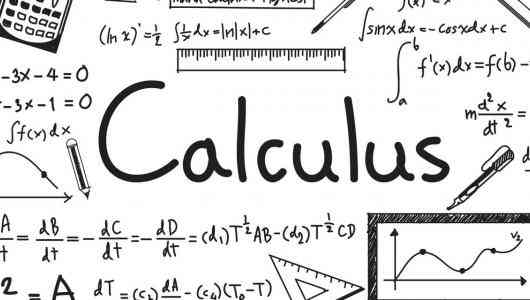# mathematics

## A collection of 6 postsscience

## Calculus: The Mathematics of Modern Age.

Calculus is the study of Change in mathematical terms, sometimes it is called "mathematics of change." It is a branch of mathematics through which we can easily explain the path of change of one variable relative to another.

You've successfully subscribed to Utopia Educators!